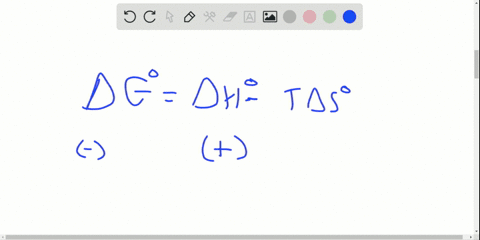Enroll in one of our FREE online STEM summer camps. Space is limited so join now!View Summer Courses### Is each statement true or false? If false, correc…

07:01Ohio State University

Need more help? Fill out this quick form to get professional live tutoring.

Get live tutoring
Problem 88

What is the change in entropy when 0.200 mol of potassium freezes at $63.7^{\circ} \mathrm{C}\left(\Delta H_{\text { fus }}=2.39 \mathrm{kJ} / \mathrm{mol}\right) ?$

Check back soon!

## Discussion

You must be signed in to discuss.

## Video Transcript

we want to calculate the change in entropy. Delta s the results in the freezing of potassium. When we freeze a substance, we go from a liquid state to a solid state. Were given a value for the temperature in the bill to each of fusion. For this process, we knew that for it face changes that the change in Gibbs free Energy Delta G will be equal to zero at constant temperatures and pressures. So now we have everything that we need from this equation to solve four Delta s And so we can rearrange that equation to form an expression four deltas be Delta G minus still to age divided by negative t. And now we plug in everything that we know. See that Delta s equals Delta G, which is again zero or face changes. Then we subtract that given Delta each of fusion 2.39 killing joules per mole. And then we divide that by the negative of the given temperature which when we converted into Kelvin, comes out to 300 36.7. And so our units for we're Delta s. The change in entropy will be killing joules per mole times, Kelvin. And if we want to play our answer by 1000 we can get it in units of jewels per mole. Kelvin, when we do that, we see we get about 7.10 Jules for more times. Kelvin. But remember, this is for the freezing of one mole of potassium. And in this problem, we're interested in the changing entropy for the freezing of 0.2 moles of potassium. So in order to find that total change in entropy, we multiply the change in entropy for one mole by that quantity and 0.2 moles that zero point 200 moles times 7.10 Jules, her mole times killed in So the units of moles cancel out. So we're left with total change in entropy units of jewels per kelvin, we get a final answer of Delta s equals 1.4 Jules Per Kelvin